# US GDP Growth Rate by Region, Second Quarter 2019

Dec 6, 2019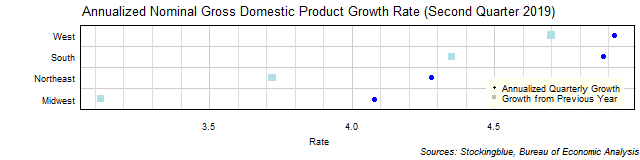The chart above shows the annualized nominal gross domestic product (GDP) quarterly growth rate in each US region as of the second quarter of 2019 and the growth rate from one year prior.  Every single region's economy grew both over the past quarter and the past year.

# EU GDP Growth Rate by State, Second Quarter 2019

Dec 3, 2019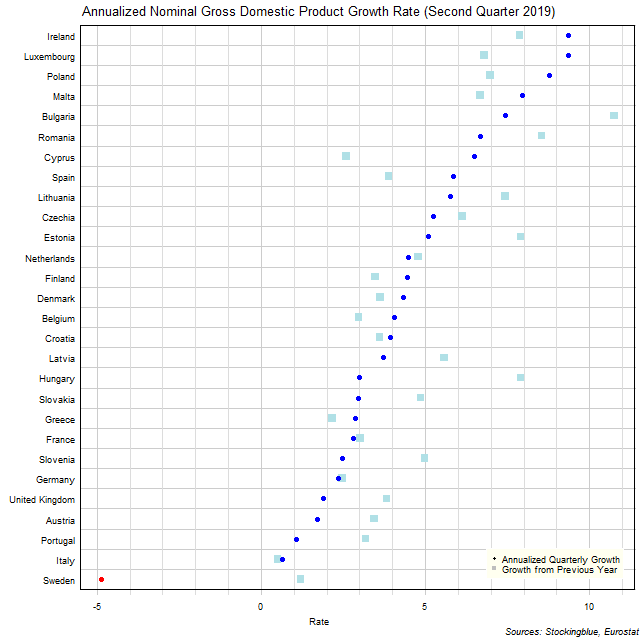The chart above shows the annualized nominal gross domestic product (GDP) quarterly growth rate in each EU state as of the second quarter of 2019 and the growth rate from one year prior.  Sweden was the only state to see a contraction over the previous quarter.

# US GDP Growth Rate by State, Second Quarter 2019

Dec 2, 2019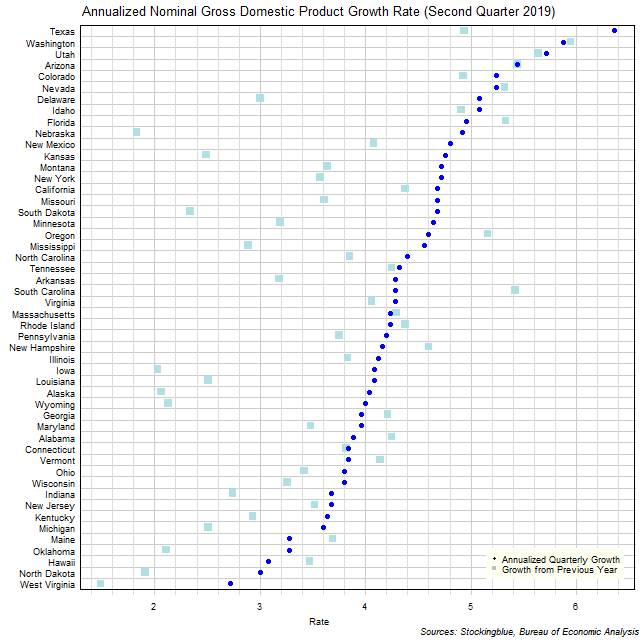The chart above shows the annualized nominal gross domestic product (GDP) quarterly growth rate in each US state as of the second quarter of 2019 and the growth rate from one year prior.  All states grew over the previous quarter and the same quarter last year.

# Per Capita GDP by EU Region, Second Quarter 2019

Nov 28, 2019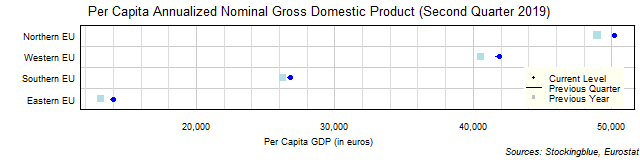The chart above shows the per capita annualized nominal gross domestic product (GDP) in each EU region as of the second quarter of 2019 in euros, the change from the previous quarter, and the per capita GDP one year prior.  Every single region's economy grew both over the past quarter and the past year.

# Per Capita GDP by US Region, Second Quarter 2019

Nov 27, 2019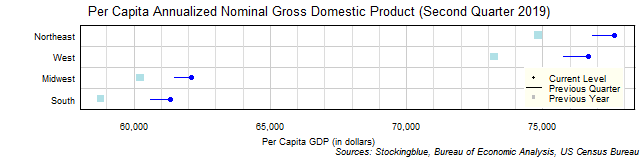The chart above shows the per capita annualized nominal gross domestic product (GDP) in each US region as of the second quarter of 2019 in dollars, the change from the previous quarter, and the per capita GDP one year prior.  Every single region's economy grew both over the past quarter and the past year.

# Per Capita GDP by EU State, Second Quarter 2019

Nov 25, 2019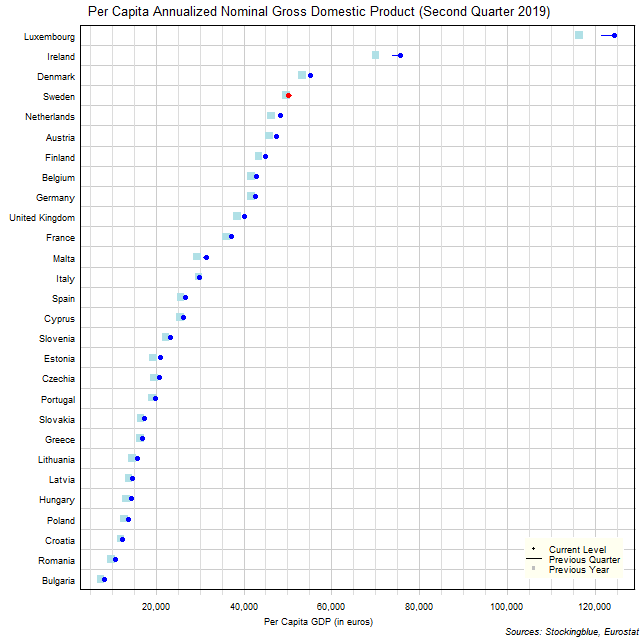The chart above shows the per capita annualized nominal gross domestic product (GDP) in each EU state as of the second quarter of 2019 in euros, the change from the previous quarter, and the GDP one year prior.  Sweden was the only state to see a drop in per capita GDP over the previous quarter.

# Per Capita GDP by US State, Second Quarter 2019

Nov 22, 2019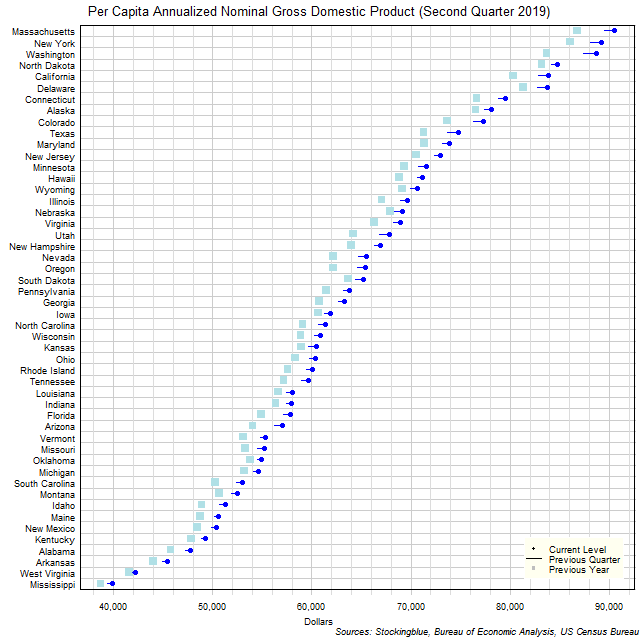The chart above shows the per capita annualized nominal gross domestic product (GDP) in each US state as of the second quarter of 2019 in dollars, the change from the previous quarter, and the GDP one year prior.  Every single state's per capita GDP grew both over the past quarter and the past year.

# EU GDP by Region, Second Quarter 2019

Nov 20, 2019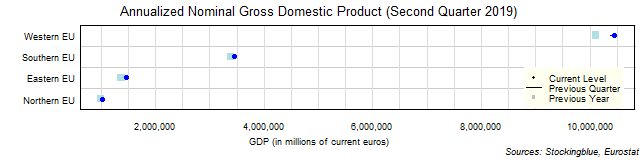The chart above shows the annualized nominal gross domestic product (GDP) in each EU region as of the second quarter of 2019 in millions of euros, the change from the previous quarter, and the GDP one year prior.  Every single region's economy grew both over the past quarter and the past year.

# US GDP by Region, Second Quarter 2019

Nov 19, 2019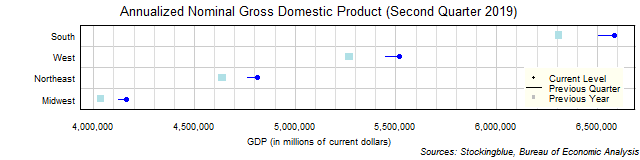The chart above shows the annualized nominal gross domestic product (GDP) in each US region as of the second quarter of 2019 in millions of dollars, the change from the previous quarter, and the GDP one year prior.  Every single region's economy grew both over the past quarter and the past year.

# EU GDP by State, Second Quarter 2019

Nov 15, 2019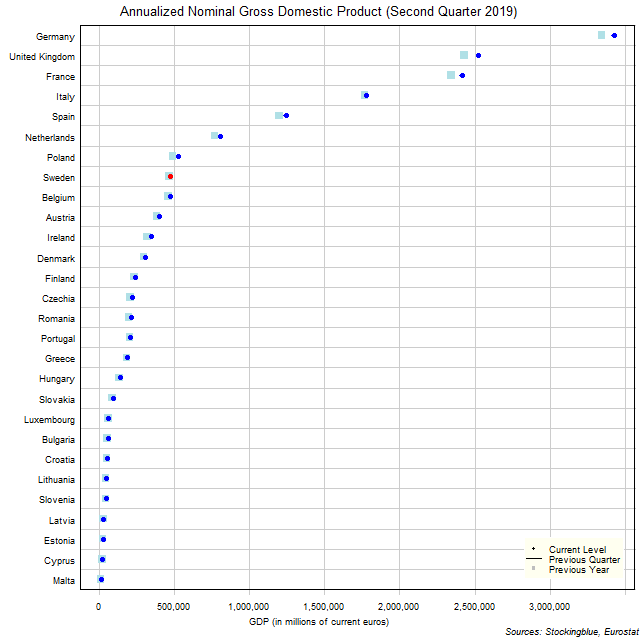The chart above shows the annualized nominal gross domestic product (GDP) in each EU state as of the second quarter of 2019 in millions of euros, the change from the previous quarter, and the GDP one year prior.  Sweden is the only state to see a drop from the previous quarter.

Older